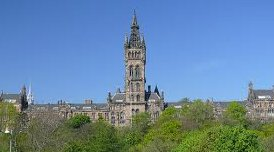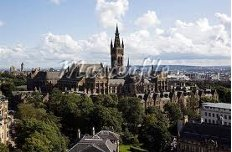Four different views of Glasgow University Tower

# Two-graphs

The files that appear below can be regarded as updated versions of some of the tables that appeared in . The additional material concerns two-graphs on 10 vertices.

The files two-graph.04, two-graph.05, two-graph.06, two-graph.07, two-graph.08, two-graph.09 (132Kb) and two-graph.10 (2.4Mb), contain tables of two-graphs on 4, 5, 6, 7, 8, 9 and 10 vertices, respectively. The format of the tables is the same as that referred to above . However, since these tables were generated by the author independently there is not an exact correspondence between the graphs in the tables. Occasionally I have listed graphs complementary to those of . Each row comprises the upper half of the (+,-) adjacency of a descendant (with its first row deleted), the eigenvalues, the order of the automorphism group, the number of graphs in the switching class, and if a two-graph is self-complementary this is denoted by the letter S. Alternatively, you may wish to download all these graphs as a gzipped tar file two-graph.tar.gz, which is around 500K.

 F.C. Bussemaker, R. A. Mathon , J.J. Seidel, "Tables of Two-graphs", Technical Report 79-WSK-05, Technical University of Eindhoven